Question

# Write the proper boundary conditions for S-Polarization and compare it with P-Polarization for linear medium.

Write the proper boundary conditions for S-Polarization and compare it with P-Polarization for linear medium.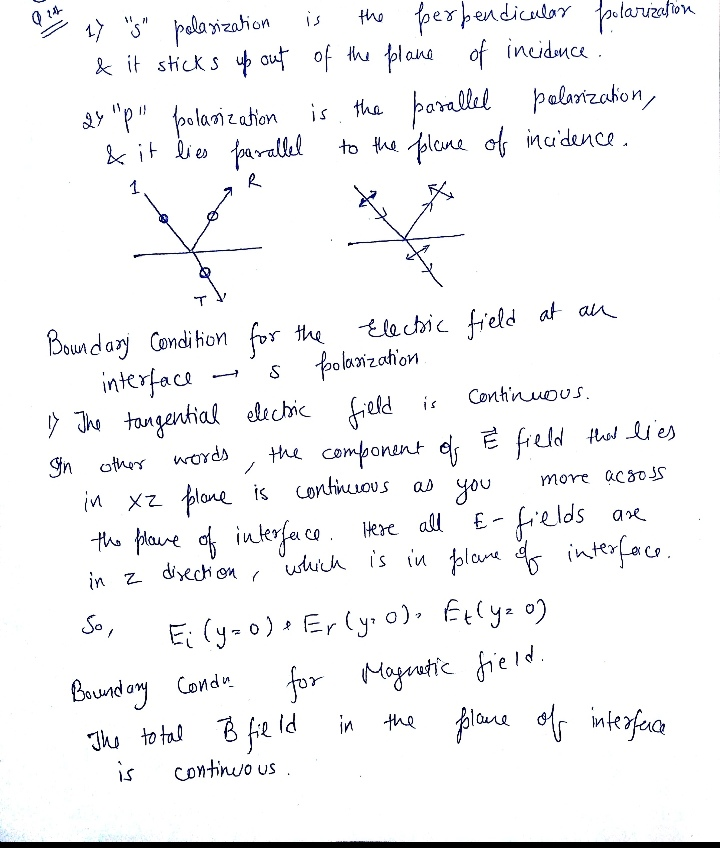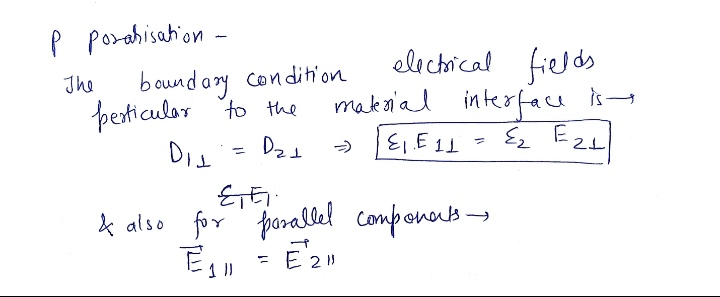thank you.

#### Earn Coins

Coins can be redeemed for fabulous gifts.

Similar Homework Help Questions
• ### 1.What is Boundary conditions for Electric potential(V) at Two dielectric material(epsilon_r1, epsilon_r2)'s boundary? Use these Boundary...

1.What is Boundary conditions for Electric potential(V) at Two dielectric material(epsilon_r1, epsilon_r2)'s boundary? Use these Boundary conditions. E_t1-E_t2=0 D_n1-D_n2=0 2.What is Boundary conditions for Intensity of Polarization(vector P) at Two dielectric material(epsilon_r1, epsilon_r2)'s boundary? Use these Boundary conditions. E_t1-E_t2=0 D_n1-D_n2=0

• ### Consider a linear operator, 82 with Po(x) pi(a) 1 p()-0 As a linear space of functions where L is self-adjoint, consider the following "periodic'-like" boundary conditions, where, as...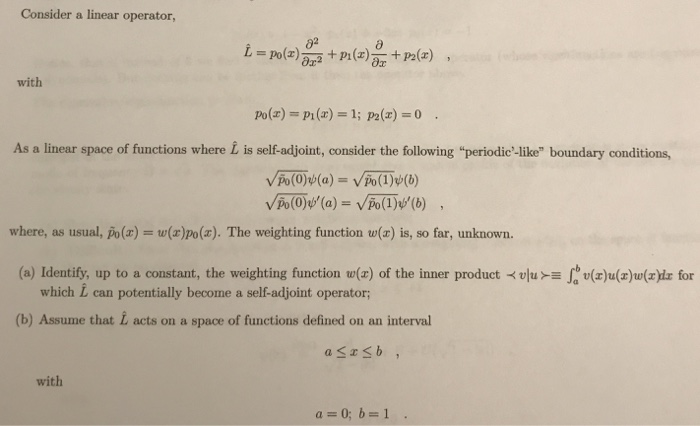Consider a linear operator, 82 with Po(x) pi(a) 1 p()-0 As a linear space of functions where L is self-adjoint, consider the following "periodic'-like" boundary conditions, where, as usual, po(z) = w(z)po(x). The weighting function w(z) is, so far, unknown. (a) Identify, up to a constant, the weighting function (a) of the inner productu for which L can potentially become a self-adjoint operator; (b) Assume that L acts on a space of functions defined on an interval with b) Show...

• ### (a) For a linear dielectric, the polarization P can be written in terms of the electric...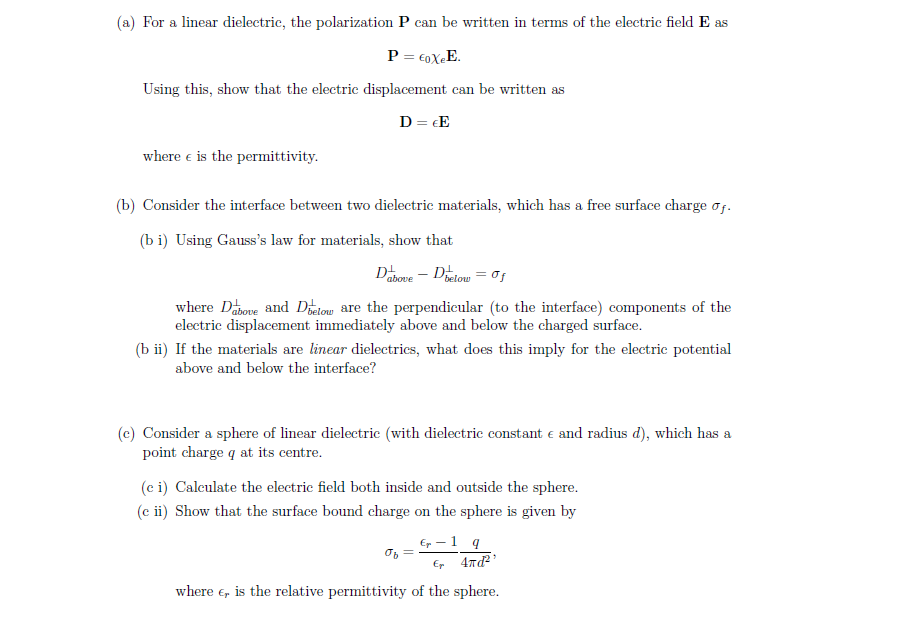(a) For a linear dielectric, the polarization P can be written in terms of the electric field E as P = EoXE. Using this, show that the electric displacement can be written as D= EE where e is the permittivity. (b) Consider the interface between two dielectric materials, which has a free surface charge of. (bi) Using Gauss's law for materials, show that Dabove – Debelow = of where Dabove and Dbelow are the perpendicular (to the interface) components of...

• ### An earthquake P wave traveling at 8.0 km/s strikes a boundary within the Earth between two...

An earthquake P wave traveling at 8.0 km/s strikes a boundary within the Earth between two kinds of material. If it approaches the boundary at an incident angle of 42 degrees and the angle of refraction is 25 degrees, what is the speed in the second medium?

• ### 2. Construct the weak form of the following linear equation. Are the boundary conditions “essenti...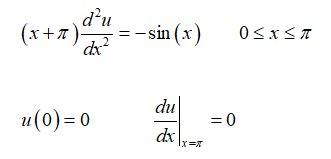2. Construct the weak form of the following linear equation. Are the boundary conditions “essential” or “natural”? Il (x +r) --sin (x ) OSxst dx du ax 11 (0)=0 =0 Il (x +r) --sin (x ) OSxst dx du ax 11 (0)=0 =0

• ### A laminar boundary layer can be approximated by a velocity profile consisting in two linear segments, as shown in Fig. 2...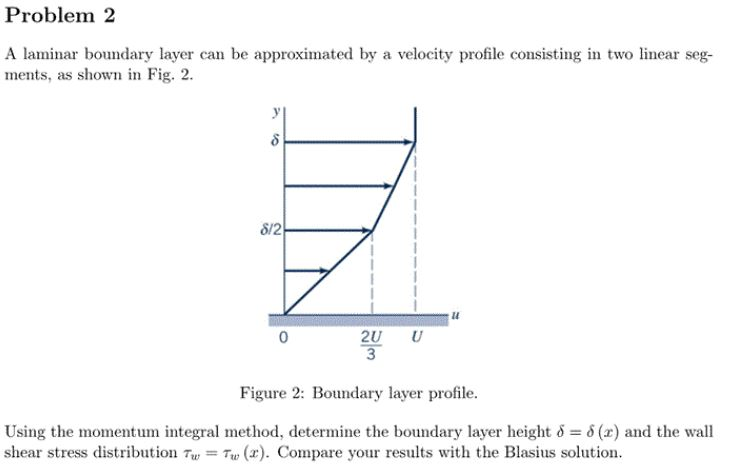A laminar boundary layer can be approximated by a velocity profile consisting in two linear segments, as shown in Fig. 2. Problem 2 A laminar boundary layer can be approximated by a velocity profile consisting in two linear seg- ments, as shown in Fig. 2 S/2 2U 3 U Figure 2: Boundary layer profile. Using the momentum integral method, determine the boundary layer height 6 (z) and the wall shear stress distribution TuTu (r). Compare your results with the Blasius...

• ### Which compound(s) can form a carboxylic acid if the proper reagent and proper conditions are chosen?...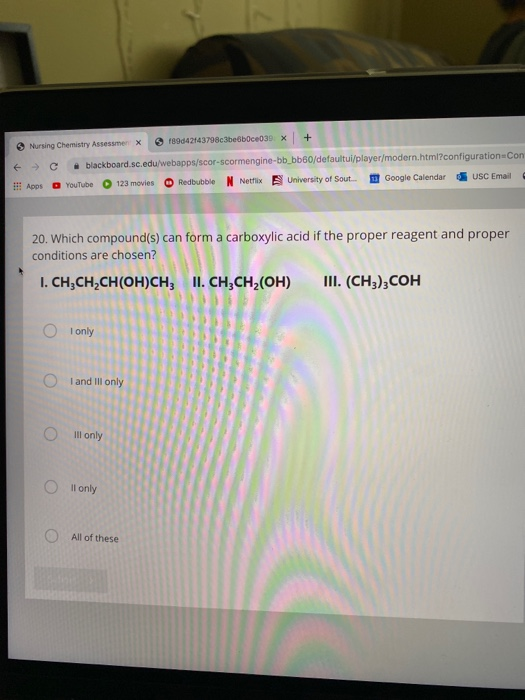Which compound(s) can form a carboxylic acid if the proper reagent and proper conditions are chosen? > Nursing Chemistry Assessmer x 189042443798c3be6b0ce039 X ( c + blackboard sc.edu/webapps/cor-sCoreTengine-bb_bb60/defaultui/player/modern.html?configuration Con I! Apps YouTube 123movies Redbubble N Netflix University of Sout. Google Calendar USC Email 20. Which compound(s) can form a carboxylic acid if the proper reagent and proper conditions are chosen? I. CH CH CH(OH)CH; II. CH3CH2(OH) III. (CH3),COH I only O and Ill only O ill only O ll only...

• ### 20. Which compound(s) can form a carboxylic acid if the proper reagent and proper conditions are...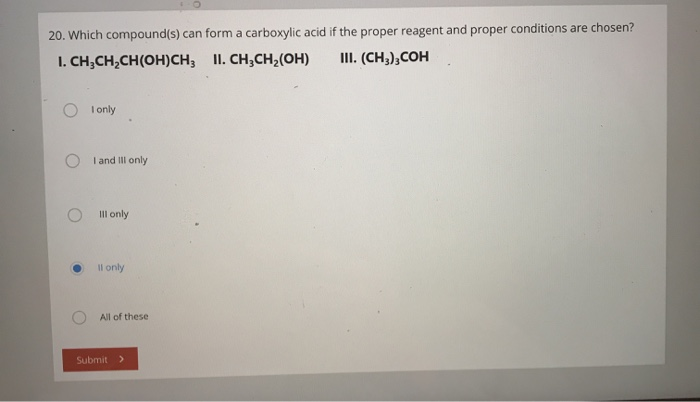20. Which compound(s) can form a carboxylic acid if the proper reagent and proper conditions are chosen? 1. CH,CH,CH(OH)CH; II. CH3CH (OH) III. (CH3),COH O only O and ill only O ill only ll only O All of these Submit >

• ### Find the intensity of reflected and transmitted waves through an interface between two linear non-conducting media...

Find the intensity of reflected and transmitted waves through an interface between two linear non-conducting media in terms of the intensity of the incident wave and the indices of refraction of the two media. Note: To do this, suppose a plane wave of frequency ω and electric field polarization in the x direction is traveling in the positive z direction. The wave approaches the interface between two media on the xy plane from left. Write complex waves equations for the...

• ### Velocity profiles in laminar boundary layers often are approximated by the equations Linear: -- U 8 lu Sin_ Sinusoidal: ร์".)-GT LU Parabolic Compare the shapes of these velocity profiles by plot...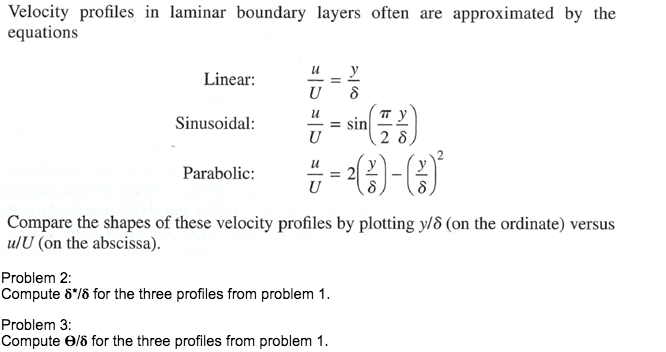Velocity profiles in laminar boundary layers often are approximated by the equations Linear: -- U 8 lu Sin_ Sinusoidal: ร์".)-GT LU Parabolic Compare the shapes of these velocity profiles by plotting y/5 (on the ordinate) versus ulU (on the abscissa). Problem 2: Compute 6 /6 for the three profiles from problem 1 Problem 3: Compute θ/6 for the three profiles from problem 1. Velocity profiles in laminar boundary layers often are approximated by the equations Linear: -- U 8 lu...